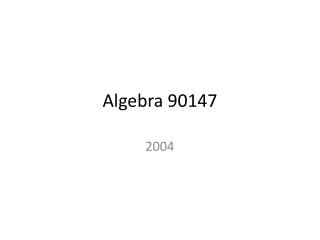# Algebra 90147 - PowerPoint PPT PresentationDownload PresentationAlgebra 90147

Algebra 90147Download Presentation## Algebra 90147

- - - - - - - - - - - - - - - - - - - - - - - - - - - E N D - - - - - - - - - - - - - - - - - - - - - - - - - - -
##### Presentation Transcript

1. Algebra 90147 2004

2. Question 1 • Solve these equations:

3. (3x – 1)(x + 2) = 0

4. (3x – 1)(x + 2) = 0

5. 6x – 2 = 2x + 9

6. 6x – 2 = 2x + 9

7. QUESTION TWO • Expand and simplify:

8. (3x – 1)(2x + 5)

9. (3x – 1)(2x + 5)

10. QUESTION THREE • Factorise completely: • x2– 7x + 6

11. QUESTION THREE • Factorise completely: • x2– 7x + 6

12. QUESTION FOUR • Simplify:

13. QUESTION FOUR • Simplify:

14. QUESTION FIVE • In a diving competition, the score (R) for a dive is calculated using the formula R = 0.6 DT • where D is the degree of difficulty for the dive and T is the total of the judges’ marks. • Jill does a dive with degree of difficulty • D = 2.5. The judges’ marks had a total T = 34.5. Calculate the score, R, for Jill’s dive.

15. QUESTION FIVE • R = 0.6 DT • D = 2.5. T = 34.5. • Calculate the score, R, for Jill’s dive.

16. QUESTION SIX • Simplify:

17. QUESTION SIX • Simplify:

18. Question 7 • John saved \$4000 for a trip to the Olympic Games.He wanted to buy as many tickets to the swimming as possible. Each ticket to the swimming costs \$85.Travel, food and accommodation cost \$3100. • Use this information to write an equation or inequation.Solve your equation or inequation.What is the greatest number of tickets to the swimming that John could buy?

19. Question 7 • John saved \$4000 for a trip to the Olympic Games. Total ≤ 4000He wanted to buy as many tickets to the swimming as possible. Each ticket to the swimming costs \$85. 85 x number • Travel, food and accommodation cost \$3100 +3100. • Use this information to write an equation or inequation.

20. Question 7

21. QUESTION EIGHT • Janet bought tickets to the diving and the swimming at the Olympic Games. She paid \$1095 for 15 tickets.The tickets for the diving cost \$65 and the tickets for the swimming cost \$85. • Solve the pair of simultaneous equations to find the number of tickets Janet bought for the swimming.

22. QUESTION EIGHT • Janet bought tickets to the diving and the swimming at the Olympic Games. She paid \$1095 for 15 tickets.The tickets for the diving cost \$65 and the tickets for the swimming cost \$85. • Solve the pair of simultaneous equations to find the number of tickets Janet bought for the swimming.

23. Cost of tickets 65d + 85w = 1095 • Number of ticketsd + w = 15

24. Use your calculator • Cost of tickets 65d + 85w = 1095 • Number of ticketsd + w = 15 • Diving = 9 • W= swimming = 6

25. OR • 65d + 85w = 1095 • 65d + 65w = 975 • 20w = 120 • w = 6

26. QUESTION NINE • At the Olympic Games 40 years ago, the average number of competitors per sport was 5 times the number of sports played.In 2004 there were 10 more sports than there were 40 years ago.In 2004 the average number of competitors per sport was 3.5 times greater than 40 years ago. • At the 2004 Olympic Games there were 10 500 competitors. Write at least ONE equation to model this situation. • Use the model to find the number of sports played at the Olympic Games 40 years ago.

27. QUESTION NINE • At the Olympic Games 40 years ago, the average number of competitors per sport was 5 times the number of sports played.In 2004 there were 10 more sports than there were 40 years ago.In 2004 the average number of competitors per sport was 3.5 times greater than 40 years ago. • At the 2004 Olympic Games there were 10 500 competitors. Write at least ONE equation to model this situation. • Use the model to find the number of sports played at the Olympic Games 40 years ago = x.

28. QUESTION NINE • At the Olympic Games 40 years ago, the average number of competitors per sport was 5 times the number of sports played. C = 5x • In 2004 there were 10 more sports than there were 40 years ago.X + 10 • In 2004 the average number of competitors per sport was 3.5 times greater than 40 years ago. C = 3.5 x 5x • At the 2004 Olympic Games there were 10 500 competitors. Write at least ONE equation to model this situation. • Use the model to find the number of sports played at the Olympic Games 40 years ago = x.

29. 10500=(x+10)×3.5×5x • 0=x2 +10x–600 • (x+30)(x–20)=0 x=20,–30 • Number of sports = 20